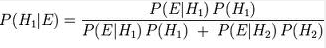## Thursday, April 18, 2013

### Note: This post represents the synthesis of the thoughts, procedures and experiences of others as represented in the 8 articles read in advance (see previous posts) and the discussion among the students and instructor during the Advanced Analytic Techniques class at Mercyhurst University in April 2013 regarding Bayesian Probability Theory specifically. This technique was evaluated based on its overall validity, simplicity, flexibility and its ability to effectively use unstructured data.

Description:
According to Hubbard, Bayesian Theory is a relationship of probabilities and “conditional” probability (Hubbard, 2010). Bayesian Analysis allows for the addition of new information as it is learned to decrease uncertainty in the hypothesis. This method allows a quantitative value to be applied to intelligence questions (ISBA, 2009). Additionally, it is very effective in reducing uncertainty.

Strengths:
1. Applicable to the intelligence field because it gives a probability of likelihood rather than statistical significance, such as in frequentist statistics.
3. Allows you to move out of the confirmation bias mindset by asking questions such as what would I observe if X were true or what would I expect to see if X were true?
4. Effective when odds are very high or very low.
5. Is more intuitive than traditional frequentist statistics.

Weaknesses:
1. Less helpful when initial probability is close to 50/50.
2. A piece of evidence that may may not be accounted for may turn out to be the most important.
3. It is difficult to decide how my weight to put into each new piece of evidence.
4. Sometimes difficult to see the real-world application and relevance.
5. Possibly time consuming to conduct bayesian theory to situations.
6. Can sometimes be fairly complex and difficult to understand.

Step by Step Action:
1. Find a topic for which you want to estimate a probability.
2. Assign probabilities to each hypothesis.
3. Update the equation by adding new hypothesis when new evidence is available.
4. Calculate the likelihood a certain scenario will occur based on the probabilities of each hypothesis.
5. For the numerator, multiply the hypothesis being tested by the probability that it will occur.
6. For the denominator, multiply each individual hypothesis by the probability of that event occurring with that hypothesis and find the sum of each probability.
7. Divide the numerator by the denominator to find the final probability.

Exercise:
In class we conducted a simple Bayesian Theory problem to determine the likelihood that an employee would show up to work late by car. The formula that was used in this Bayesian approach was the general form of bayes theorem equation as shown below. There were two parts to the exercise, part A included the bosses probabilities that Bob would come late to work by car, bus, or train, overall determining the probability that Bob came to work late by car. Part B analyzed the same situation, but the probabilities of how a co-worker thought Bob would come late to work by car. By calculating both Part A and Part B it was noticed how the probabilities of Bob coming to work late by car changed with different evidence placed into the general form bayesian equation. In terms of real world applicability, the exercise demonstrated how applying bayesian theory to real life scenarios may be difficult to initiate or not seem as the best approach to figuring out a situation. It would seem just asking Bob what mode of transportation he came to work late in would be a much easier approach. However, the exercise does demonstrate the benefits of how bayesian theory can reduce the uncertainty of a question when more evidence is added, which is essential in the work conducted by intelligence analysts. Overall, Bayesian Theory can provide a much more reliable estimate for the analyst.